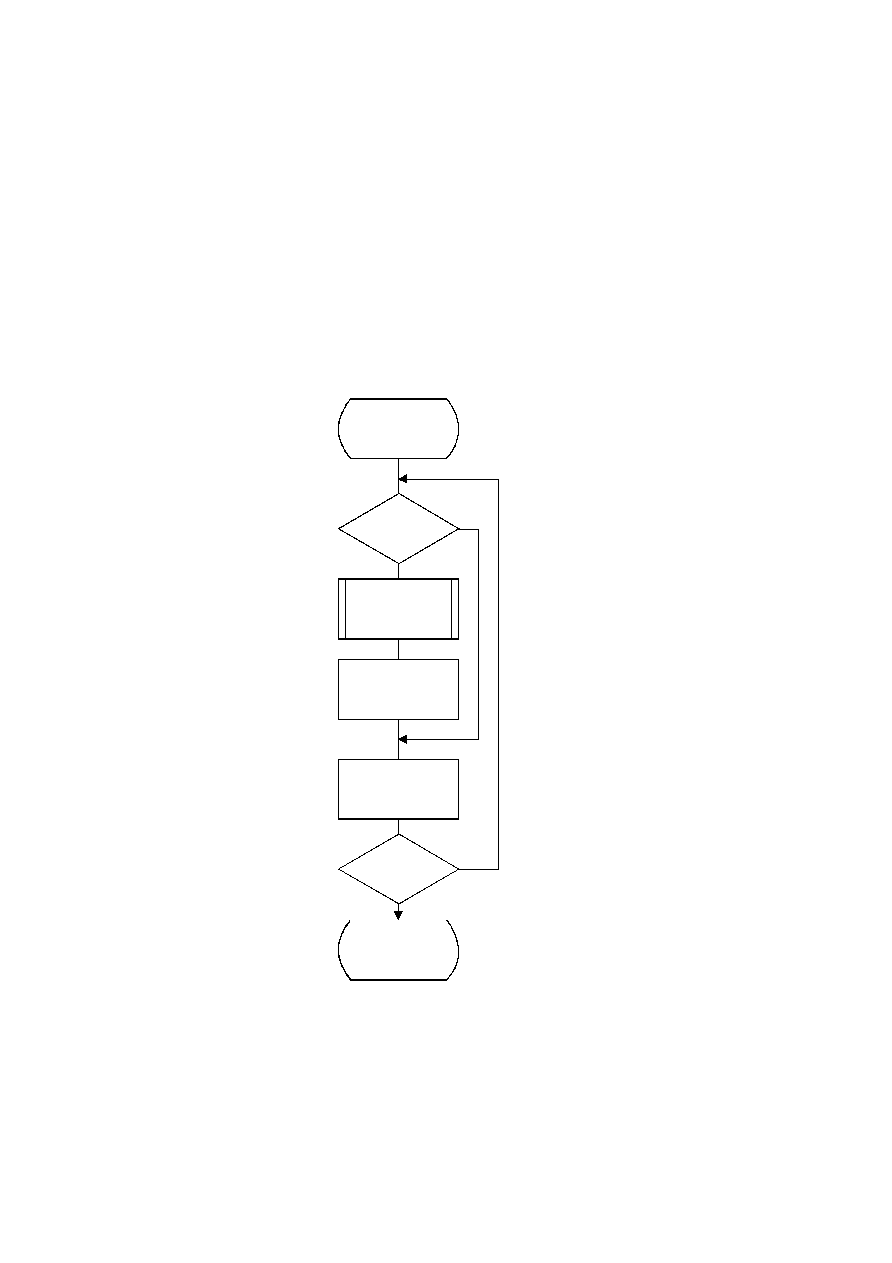ISO/IEC 10918-1 : 1993(E)
D.2.5
Probability estimation in the decoder
The procedures defined for obtaining a new LPS probability estimate in the encoder are also used in the decoder.
D.2.6
Renormalization in the decoder
The Renorm_d procedure for the decoder renormalization is shown in Figure D.19. CT is a counter which keeps track of
the number of compressed bits in the C-low section of the C-register. When CT is zero, a new byte is inserted into C-low
by the procedure Byte_in and CT is reset to 8.
Both the probability interval register A and the code register C are shifted, one bit at a time, until A is no longer less than
X'8000'.
TISO1190-93/d057
Renorm_d
Yes
No
Byte_in
CT = 8
Done
Yes
No
A = SLL A 1
C = SLL C 1
CT = CT ­ 1
CT = 0
?
A < X'8000'
?
Figure D.19 ­ Decoder renormalization procedure
Figure D.19 [D57], = 16.5 cm = 645.%
CCITT Rec. T.81 (1992 E)
73